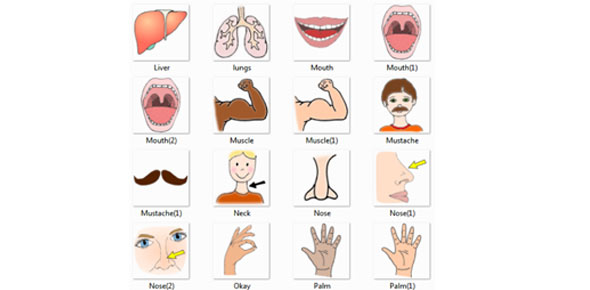G12 Right Hand Rule

10 Questions | Total Attempts: 72Settings• 1.
To create a force, the magnetic field and the current have to be parallel
• A.

True

• B.

False

• 2.
What is the direction of the force?
• A.

Up

• B.

Down

• C.

Right

• D.

Left

• 3.
Which diagram shows the correct shape of the field?
• A.

A

• B.

B

• C.

C

• D.

D

• 4.
Where is the north pole created?
• A.

Left

• B.

Right

• 5.
The magnetic field generated by a current into (X) the screen is...
• A.

Clockwise

• B.

Anticlockwise

• 6.
What is the direction of the force?
• A.

Up

• B.

Down

• C.

Right

• D.

Left

• 7.
What is the direction of the force?
• A.

Up

• B.

Down

• C.

Right

• D.

Left

• 8.
If current is passed through a loop of wire, as shown, which direction will the loop rotate?
• A.

Clockwise

• B.

Anticlockwise

• 9.
If the ends of a wire loop are attached to two half-circular metal strips, arranged so that the two strips almost form a complete circle, and those strips are contacted by two "brushes" which connect to opposite poles of a battery, what polarity of voltage will be measured as the loop is rotated counter-clockwise?
• A.

Right Postive, Left Negative

• B.

Right Negative, Left Positive

• 10.
The thumb, in the right hand rule, indicates the direction of the current
• A.

True

• B.

False

Related TopicsBack to top